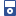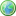Accessibility options:

# Sequences & Series resources

Show me all resources applicable to

### iPOD Video (2)Limits of sequences
Video for iPod. This resource is released under a Creative Commons license Attribution-Non-Commercial-No Derivative Works and the copyright is held by Skillbank Solutions Ltd.Sigma notation
Video for iPod. This resource is released under a Creative Commons license Attribution-Non-Commercial-No Derivative Works and the copyright is held by Skillbank Solutions Ltd.

### Teach Yourself (4)Arithmetic and Geometric Progressions
This unit introduces sequences and series, and gives some simple examples of each. It also explores particular types of sequence known as arithmetic progressions (APs) and geometric progressions (GPs), and the corresponding series.Limits of sequences
In this unit, we recall what is meant by a simple sequence, and introduce infinite sequences. We explain what it means for two sequences to be the same, and what is meant by the n-th term of a sequence. We also investigate the behaviour of infinite sequences, and see that they might tend to plus or minus infinity, or to a real limit, or behave in some other way.Sigma notation
Sigma notation is a method used to write out a long sum in a concise way. In this unit we look at ways of using sigma notation, and establish some useful rules.The sum of an infinite series
In this unit we see how finite and infinite series are obtained from finite and infinite sequences. We explain how the partial sums of an infinite series form a new sequence, and that the limit of this new sequence (if it exists) defines the sum of the series. We also consider two specific examples of infinite series that sum to e and pi respectively.

### Test Yourself (1)Maths EG
Computer-aided assessment of maths, stats and numeracy from GCSE to undergraduate level 2. These resources have been made available under a Creative Common licence by Martin Greenhow and Abdulrahman Kamavi, Brunel University.

### Video (1)Arithmetic and Geometric Progressions
This unit introduces sequences and series, and gives some simple examples of each. It also explores particular types of sequence known as arithmetic progressions (APs) and geometric progressions (GPs), and the corresponding series. (Mathtutor Video Tutorial) This resource is released under a Creative Commons license Attribution-Non-Commercial-No Derivative Works and the copyright is held by Skillbank Solutions Ltd.

Website design by Pink Mayhem, Leicester